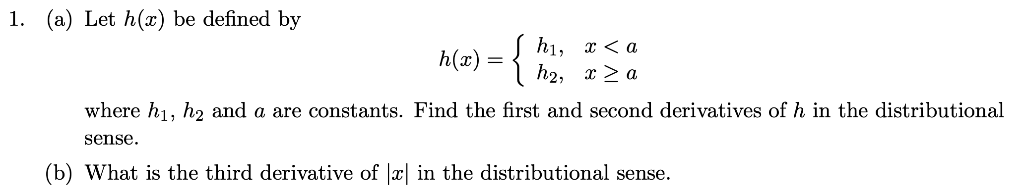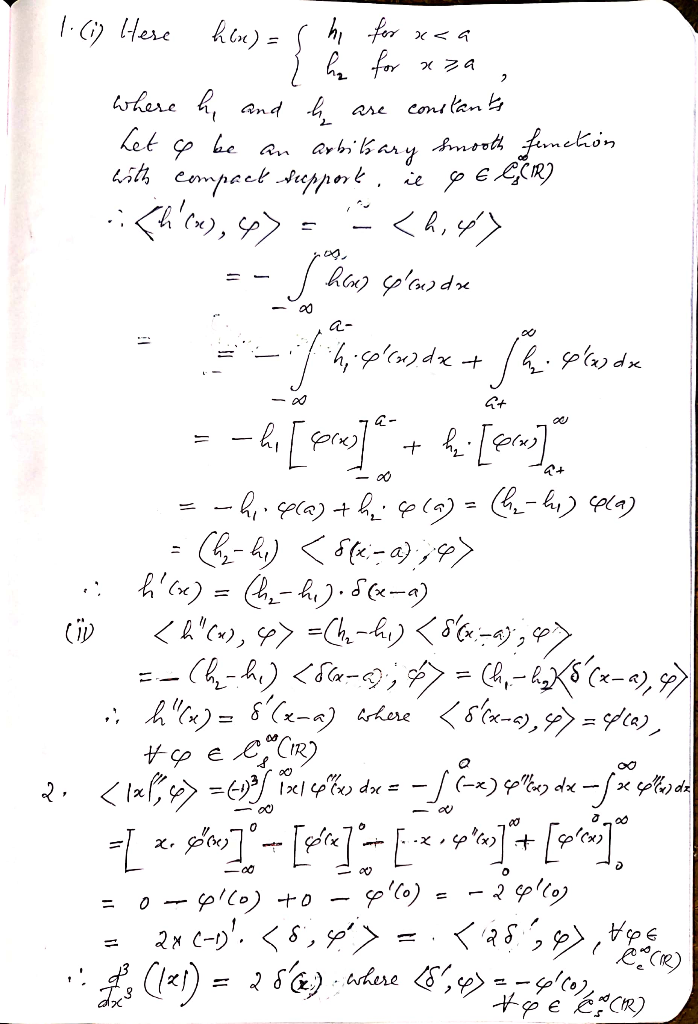# (a) Let h() be defined by 1. h(x) h2, х > а where h h2 and a are constants. Find the first and second derivatives of...(a) Let h() be defined by 1. h(x) h2, х > а where h h2 and a are constants. Find the first and second derivatives of h in the distributional sense (b) What is the third derivative of |x| in the distributional sense.##### Add Answer of: (a) Let h() be defined by 1. h(x) h2, х > а where h h2 and a are constants. Find the first and second derivatives of...
More Homework Help Questions Additional questions in this topic.